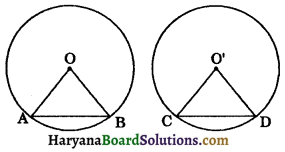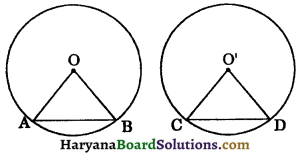# HBSE 9th Class Maths Solutions Chapter 10 Circles Ex 10.2

Haryana State Board HBSE 9th Class Maths Solutions Chapter 10 Circles Ex 10.2 Textbook Exercise Questions and Answers.

## Haryana Board 9th Class Maths Solutions Chapter 10 Circles Exercise 10.2

Question 1.
Recall that two circles are congruent if they have the same radü. Prove that equal chords of congruent circles subtend equal angles at their centres.
Solution:
Given: AB and CD are two equal chords of two congruent circles C(O, r) and C(O’, r) respectively.To prove:
∠AOB = ∠CO’D.
Proof: In ΔAOB and ΔCO’D, we have
OA = O’C [Equal radii of congruent circles]
OB = O’D [Equal radii of congruent circles]
AB = CD (given)
∴ ΔAOB ≅ ΔCO’D (By SSS congruence rule)
∠AOB = ∠CO’D (CPCT)
Hence proved.Question 2.
Prove that if chords of congruent circles subtend equal angles at their centres, then the chords are equal.
Solution:
Given: AB and CD are two chords of congruent circles C(O, r) and C(O’, r) such that ∠AOB = ∠CO’D.To prove : AB = CD.
Proof: In ΔAOB and ΔCO’D, we have
AO = O’C [Equal radii of congruent circles]
∠AOB = ∠CO’D (given)
OB = O’D [Equal radii of congruent circles]
∴ ΔAOB ≅ ΔCO’D [By SAS congruence rule]
⇒ AB = CD (CPCT)
Hence proved.